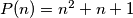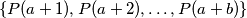### IMO Shortlist 2016 problem N3

Kvaliteta:
Avg: 0,0
Težina:
Avg: 7,0

A set of postive integers is called fragrant if it contains at least two elements and each of its elements has a prime factor in common with at least one of the other elements. Let$P(n)=n^2+n+1$. What is the least possible positive integer value of$b$ such that there exists a non-negative integer$a$ for which the setis fragrant?

Izvor: https://www.imo-official.org/problems/IMO2016SL.pdf